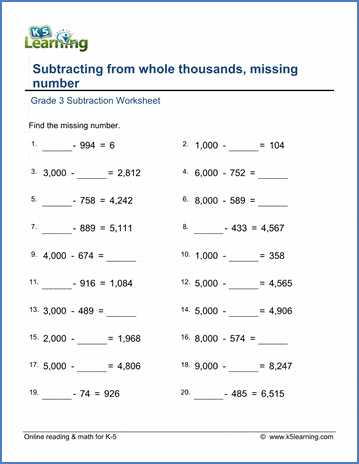# Subtract from thousands, missing number

## Worksheets: Subtracting from whole thousands with missing numbers

Below are six versions of our grade 3 math worksheet on subtracting a 3-digit number from whole thousands. The minuend (the first number in the subtraction equation) is always a whole thousand (1,000 - 9,000) from which random numbers are subtracted. Students are asked to fill in the missing number - a challenging mental math exercise! These worksheets are pdf files.## More subtraction worksheets

Explore all of our subtraction worksheets, from subtracting by counting objects to subtracting large numbers in columns.

## What is K5?

K5 Learning offers reading and math worksheets, workbooks and an online reading and math program for kids in kindergarten to grade 5.  We help your children build good study habits and excel in school.